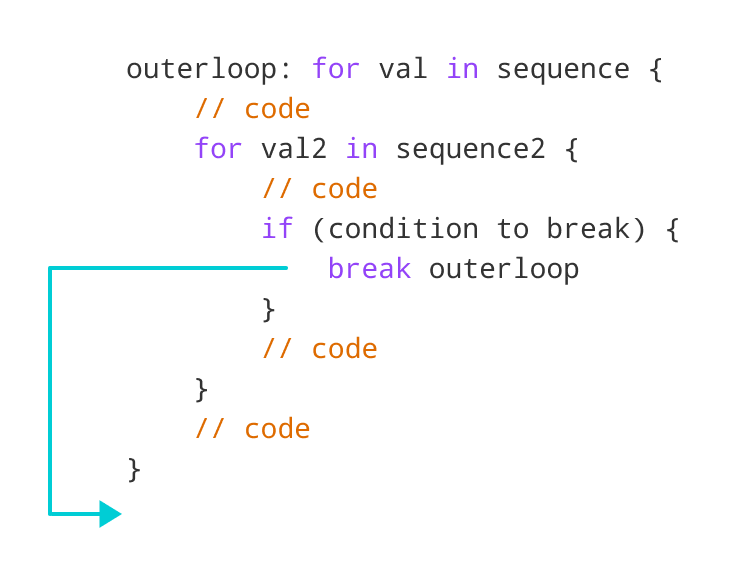# Swift break Statement

In this tutorial, you will learn about the break statement with the help of examples.

The `break` statement is used to terminate the loop immediately when it is encountered.

The syntax of the `break` statement is:

``break``

Before you learn about the `break` statement, make sure you know about:

## Swift break statement with for loop

We can use the `break` statement with `for` loop to terminate the loop when certain condition is met. For example,

``````for i in 1...5 {

if i == 3 {
break
}

print(i)
}``````

Output

```1
2```

In the above example, we have used the `for` loop to print the value of `i`. Notice the use of the `break` statement,

``````if i == 3 {
break
}``````

Here, when `i` is equal to 3, the `break` statement terminates the loop. Hence, the output doesn't include values after 2.

Note: The `break` statement is almost always used with decision-making statements.

## break with while Loop

We can also terminate a `while` loop using the `break` statement. For example,

``````// program to find first 5 multiples of 6

var i = 1

while (i<=10) {
print("6 * \(i) =",6 * i)

if i >= 5 {
break
}

i = i + 1
}``````

Output

```6 * 1 = 6
6 * 2 = 12
6 * 3 = 18
6 * 4 = 24
6 * 5 = 30```

In the above example, we have used `while` loop to find the first 5 multiples of 6. Here notice the line,

``````if i >= 5 {
break
}``````

This means when `i` greater than or equal to 5, the `while` loop is terminated.

## Swift break statement with nested loops

We can also use the `break` statement inside a nested loop.

• If we use `break` inside the inner loop, then the inner loop gets terminated.
• If we use `break` outside the inner loop, then the outer loop gets terminated.
``````// outer for loop
for i in 1...3 {

// inner for loop
for j in 1...3 {

if i == 2 {
break
}

print("i = \(i), j = \(j)")
}
}``````

Output

```i = 1, j = 1
i = 1, j = 2
i = 1, j = 3
i = 3, j = 1
i = 3, j = 2
i = 3, j = 3 ```

In the above example, we have used the `break` statement inside the inner `for` loop.

``````if i == 2 {
break
}``````

Here, when value if `i` is `2`, the `break` statement is executed. It terminates the inner loop and control flow of the program moves to the outer loop.

Hence, the value of `i = 2` is never displayed in the output.

## Swift Labeled break

Till now, we have used the unlabeled `break` statement. However, there's another form of `break` statement in Swift known as the labeled break.

When using nested loops, we can terminate the outer loop with a labeled break statement.Working of the Swift Labeled break Statement

As you can see in the above image, we have used the `outerloop` identifier to specify the outer loop. Now, notice how the `break` statement is used (`break outerloop`).

Here, the `break` statement is terminating the labeled statement (i.e. outer loop). Then, the control of the program jumps to the statement after the labeled statement.

### Example: Labeled Statement with break

``````outerloop: for i in 1...3{

innerloop: for j in 1...3 {

if j == 3 {
break outerloop
}

print("i = \(i), j = \(j)")
}
}``````

Output

```i = 1, j = 1
i = 1, j = 2```

In the above example, we have labeled our loops as:

`outerloop: for i in 1...3 {...}`

`innerloop: for j in 1...3 {...}`

This helps to identify the loops. Notice the use of labeled `break` statement.

``````if j == 3 {
break outerloop
}``````

Here, the `break` statement will now terminate the outer loop labeled with `outerloop`.